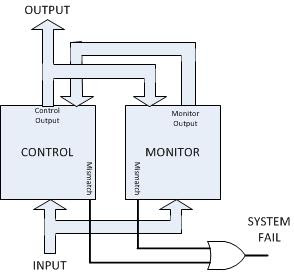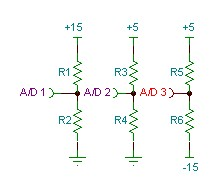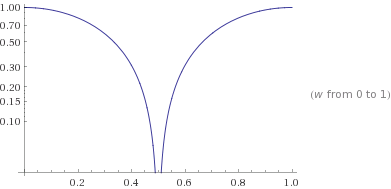## Saturday, August 4, 2018

### Cross Checking SystemsFigure 1 - Typical Cross checking system.
An interesting type of redundant system is the cross checking system.  Cross checking systems increase the system integrity.   In this system two identical computer systems run the processing in parallel.  If the processing does not exactly match, a system fail is initiated.  Cross checking systems are one way systems are designed to protect against single bit errors.
The block diagram to the left depicts a typical system.  Here are two processors, the control and the monitor processors.  The system inputs must be identically applied to both processor.  They may be sensor inputs, inputs for control, clocking,...  In a deterministic system, both of these processors should have identical outputs for identical inputs.  Both processors should calculate the same exact system output.  Both processors monitor the other processors system output.   Both processors compare their calculated output to the other processors output.  If they do not match, the output line 'Mismatch' is asserted.  The "System Fail" output is asserted if either processor declares a mismatch.  There are two interesting things to note with this system.  First is that only the output from the Control processor is actually used.  The second is that although system integrity is increased, system reliability (which is measured in FIT or MTBF) will decrease.  This is because if either processor fails, then the system fails.  Although MTBF decreases, what is gained is the knowledge that the system has failed.
In applications such as safety where integrity is important, cross checking systems are a very practical design possibility.

## Saturday, June 23, 2018

### Byzantine networks and the Byzantine Generals Problem

In some applications, networks and computer systems must be safe. Typically what we do is to use redundancy to protect against failures. If one component fails, another one takes over. As the tolerance for failure decreases, the cost and complexity of some of these systems increase. Byzantine networks study what happens when we have very little tolerance for failure. In fact, they consider what happens when one component does not just fail, but that component is malicious and tries to make the whole system fail.

The Byzantine Generals are a metaphor for a distributed network. In this metaphor the Generals are each computers networked together, each giving orders to their connected components. What happens when one General is 'malicious'. I.e. it fails in a way that it tries to take down other generals? What protocol should the generals adhere to so they don't follow a malicious generals? So the rest of the system operates normally.

Other than Byzantine networks there are other, less stringent architectures to protect against failures. These other solutions, such as redundancy and voting, relax the constraint of failure in various ways.

Following is a Link for a proposal to identify malicious players in Byzantine Network. This proposal assumes the network is a modern TCP/IP network, so 'omission' faults are identified by the underlying network. Click here to read the research proposal..

## Saturday, March 5, 2016

### Interesting Circuit Problem

A while back I ran across a circuitry problem that may interest those with a little electronics knowledge. We were getting failures on a high percentage of new product, but not on all of them. The product was warning that its internal -15v supply was out of tolerance (and faulting). A manual check with a voltmeter showed the -15v supply was within the required +/-10% tolerance. Other internal supplies were monitored as well by the computer and they never exhibited this issue.

Below is a schematic of the components involved, what I found, and the interesting solution. What this schematic is showing is three resistor divider circuits that divide down the +15, +5v and the -15 volt supplies to feed the A/D converter inputs (there is some additional filtering and protection not shown).Scaling Circuitry
This A/D converter can resolve a range of 0v to +3v signal thus the need to divide down the voltages before conversion. Additionally the -15v rail voltage is 'translated' to a positive voltage by raising it with the +5V.  The code in the computer then reads the A/D converter and looks to see if the value read is out of +/-10% tolerance.

There is a problem though with the -15v divider circuit. Do you see it? The -15v circuit is dependent on the +5v voltage as well. So for instance if the +5v rail is 8% too high (within spec), and the -15v signal is 8% too low (also within spec), the resulting divided/translated voltage may be more than 10% out of whack (this is a highly technical term). The unit might then erroneously fault. I found a simple software change that put this issue to rest. We need to first read the +5 rail voltage. Then the -15v rail voltage is read and we subtract out the component of +5v rail voltage from the -15v reading. To do this requires some math and Kirchoff's voltage law.

VAD3 = V+5v - ( V+5v - V-15v) R5 /( R5 + R6

One note on the expression parameters,  V+5v  is the actual measured voltage of the +5V supply.   This is obtained by reading the VAD2 voltage first and calculating the +5V actual voltage. Next we have to solve the equation for the unknown voltage, V-15 :

V-15v = (V+5v - VAD3)(R5 + R6)/R5 + V+5v

Everything on the right hand side is known.  I implemented this equation in the firmware of the computer and it worked like a charm! I implemented it, but did not consider this solution to be optimal. In subsequent designs I made sure we did not use the +5v supply to translate the -15v supply voltage, but for this product this software solution had several benefits:

• Easy fix for units already in field. No recall.
• No waste for already manufactured inventory. We can use existing stuffed pcb's.
• We did not need to delay an already delayed product.
• No additional cost for the pcb NRE.
There were some other issues with this solution, scaling issues and floating point issues, but those are for another lab note.

## Saturday, February 20, 2016

### Why is aliasing important to A/D converters?

To minimize aliasing an anti-aliasing filter is needed before an A/D converter. How do you design this pre-filter? What is this aliasing? Shannon, and Nyquist later, said that the maximum signal we can reconstruct is half of the sampling rate. If the signal we sample has components higher than 1/2 sampling rate then we get a phenomena called 'aliasing. If I were to send an aliased signal back out of a computer to a D/A converter it would look like the signal was 'shifted' in frequency.

Suppose a signal of Fs was put into an A/D converter and the sampling rate was Fs. It might appear on a D/A converter as a DC signal .

As an everyday example of aliasing, have you ever seen a moving fan appear still under a strobe light? When the strobe is blinking at the same rpm's as the fans rotational speed, the fan will appear motionless. The aliasing phenomena can occur if the sampled signal is at or above 1/2 the sampling rate OR IF IT HAS (Fourier) COMPONENTS AT OR ABOVE THIS RATE. In this case just those components above the Nyquist rate would be aliased, but the total signal, if reconstructed, will be distorted.

How do we design to this? Typically we use an anti-aliasing filter on the front end of the A/D. This filter is designed to attenuate signal above the Nyquist rate to below some noise level. It might be as simple as an RC or more complicated as a multi-pole active filter in a Chebychev or Butterworth configuration. The key is to understand how much attenuation we need at the stop band. Suppose our A/D is 12 bits. The ideal SNR is 72dB (= 20* log(4096)). The filter should be designed to provide 72 db of attenuation at the stop band. Now we note that a single pole filter provides 6db per octave of attenuation.

A single pole low pass filter designed to attenuate a signal to this noise specification would be designed to have a cutoff 12 octaves below half the A/D sampling rate!

## Sunday, February 14, 2016

### How to find a short on a PCB

When I am troubleshooting new PCB's I have a unique technique for finding shorts on PCB's. I actually have to hand the credit for this technique to a business partner, Dr. Ed Kafrissen. He came up with this idea.  So let me set up a scenario for you, and then show you Ed's solution. Suppose you have a client and you need to deliver 100 PCB's to him/her quickly. You have someone stuff the boards for you, now you have to verify they work.

One of the first tests we would do is to apply power to the new PCB and bring the voltage up slowly. Perhaps without any chips installed. If the power supplies load down, then you got a problem to solve. You got a short on the PCB.Ed came up with simple and unique solution. He would current limit a power supply to some small current, and at the rated voltage of the pcb. Ed would then hook up the PCB, which immediately loaded down the current limited supply. He would turn up the current on the supply as he felt on the PCB for a hot spot! Invariably some IC would be stuffed in backwards that our visual inspection did not find, or we would get a PCB which was not completely etched.  Alternatively, you can use a handheld IR imaging thermometer to pinpoint the hot-spot.

Anyway, that was Ed's solution to quickly find shorts on a PCB...and it worked well as long as you were careful enough to not burn yourself! Yeow!

## Sunday, February 7, 2016

### The Exponential Digital Lowpass Filter

In the last lab note we looked at the boxcar filter structure. That was an example of an FIR filter. The exponential filter is an IIR filter.  If I need a little filtering I usually use this filter structure. In a simple implementation of this filter we calculate the filter output by taking the current sample, adding it to the previous output then dividing by 2. Mathematically y=(x+yz-1) ; where y is the current output, x is the current input, and yz-1is the previous output.  The z-1 term shifts back in time 1 sample period.  Since the output is a function of the previous output this is an Infinite Impulse Response filter, or IIR filter.

In a typical embedded system we work with a fixed point math.

For the above function the characteristic equation is H(z) = Y/X = 1/(2-z-1)= z/(2z-1); There is a zero at 0 and a single pole at z=1/2. This is within the unit circle, thus the filter is stable. To plot the frequency response we set z=ejωT. Then we normalize by setting T=1. We can use Wolfram Alpha to plot the equation H(ω) = 1/(2-e-jω) . Click this link to see plot.  I copied the plot here for convenience.Exponential Lowpass Filter response.

Here the horizontal axis is in Hz This filter attenuates to 0.7 at a frequency of 0.14Hz (remember this equation is normalized to a sampling frequency of 1 Hz).

If this is too much filtering, or too little filtering you can try adjusting the weighting of the two terms in the exponential filter. For instance, what if you choose the weighting y = ¼ (3x + yz-2)?  Here I tried to use a power of 2 divisor to make the math dead nuts simple to implement on a fixed point processor. On an FPGA you would not even need to divide or shift, just pick off the right bits. The FPGA would need just two 'add' operations. Can you calculate the frequency domain function and plot it?

## Sunday, January 31, 2016

### The Boxcar Digital Lowpass Filter

In embedded systems it is quite common to need a little bit of filtering on analog quantities. Programmers sometimes feel they need the filtering to help smooth any analog conversion noise. Two type of filters commonly used are the Boxcar and the Exponential filter. Both are simple to implement. Its important to understand the impact these filters have on delay and frequency response, and to evaluate their comparative effectiveness.

The Boxcar filter is a simple averaging filter. Technically it is an FIR filter. Typically you would obtain your next analog value, add it to the last n-1 values read, then divide by n. It's just a simple average of the last few values read.

A common value for n is 4. The Z transform of a filter with n=4 is H(z) = ¼ (z3+z2+z+1)/(z3) . There are three poles at the origin in the Z domain. Thus this filter is stable. There is one real zero at -1, and two complex conjugate zero's at +i and -i. The (normalized) frequency response is given by H(e) = magnitude(¼ + ¼e-jω+ ¼e-j2ω+¼e-j3ω).
Click on the link to use Wolfram-Alpha to plot this function. In the following image (copied from Wolfram) we see several cycles of normalized frequency response including mirroring above half the sample rate.   The mirroring is how aliasing shows up in the frequency response.Box Car Filter Normalized Response.  N=4.

Note that the horizontal axis is in Hz. The sampling frequency is 1Hz.  (The response is normalized to 1 Hz.)  One half the sample rate is 0.5Hz - the Nyquist rate. What you are seeing above 0.5Hz is a periodic mirror of the response. But lets focus on the response below 0.5 Hz.  How UGLY for a low pass response.  Lets try to plot the response for n=2. With this filter all we do is add the current and previous samples and divide by 2. The characteristic equation is Y = ½(X + Xz-1) .  The transfer function is H(z) = ½(1+z-1) = ½(1+z)/z . This has a single pole at 0 and a zero at -1. Thus its stable. The normalized frequency domain function H(e) = ½(1+e-jω).  Its normalized for a sample time of 1sec. Click here to have Wolfram Alpha plot the response.Box Car Filter Normalized Response.  N=2.
This looks more like a low pass response. Note that the horizontal axis this time is in Hz. Since the sample time is a normalized 1second, then the sample frequency is 1.0 Hz, and the Nyquist frequency is 0.5 Hz.

In my next Laboratory Note we will look at the Exponential Filter. This is actually my favorite and I will show you why!

## Sunday, January 24, 2016

### 3D web graphics.

A new feature of modern web browsers is web.gl.  This language allows browsers to take advantage of the hardware graphics acceleration in your computer.  Its a little awkward to program in so the three.js library was born.  Its an open source project.  To learn this framework I created some experiments.  Please click on the links below.  The images can be rotated and panned with your mouse.

A starburst pattern   A cylinder made of lines
A flat Square   An open cube with shading
Polygon with gradient coloring - these were some simple, first experiments

Stairway with Shading       Mesh a Cylinder
Mesh a segmented Cylinder
Mesh a Cylinder turned into a vase
Mesh a Cylinder turned into a torus - these are meshed from many tiny, individual
triangles. They do not use the Three.js premeshed objects.

Cube matrix with animated color    An animated solar system
Bunch of Boxes   A ziggurat
A ziggurat with controls
A helix of objects  A helix of any 'ole object

Citi-scape
Cubes changing color      A tiny solar system
A torus explorer   Click square to make wave

Finally, these are some water simulations.  Click on the water to make waves.:
Hugo Elias Algorithm - makes multiple waves with interference at edges
Transverse Wave Algorithm - can make 1 wave at a time.
Another algorithm